Disclaimer: This is an example of a student written essay.

Any opinions, findings, conclusions or recommendations expressed in this material are those of the authors and do not necessarily reflect the views of UKEssays.com.

# Detecting Complex Image Data Using Data Mining Techniques

 ✅ Paper Type: Free Essay ✅ Subject: Computer Science ✅ Wordcount: 3498 words ✅ Published: 26th Mar 2018

Detecting complex image data using data mining techniques

IMRAN KHAN

ABSTRACT

The Internet, computer networks and information are vital resources of current information trend and their protection has increased in importance in current existence. The intrusion detection system (IDS) plays a vital role to monitors vulnerabilities in network and generates alerts when found attacks. Today the educational network services increasing day today so that IDS becomes essential for security on internet. The Intrusion data classification and detection process is very complex process in network security. In current network security scenario various types of Intrusion attack are available some are known attack and some are unknown attack. The attack of know Intrusion detection used some well know technique such as signature based technique and rule based technique. In case of unknown Intrusion attack of attack detection is various challenging task. In current trend of Intrusion detection used some data mining technique such as classification and clustering. The process of classification improves the process of detection of Intrusion. In this dissertation used graph based technique for Intrusion classification and detection. This dissertation proposes efficient intrusion detection architecture which named IDS using improved ensemble techniques (IDSIET). The IDSIET contains a new improved algorithm of attribute reduction which combines rough set theory and a method of establishing multiple rough classifications and a process of identifying intrusion data. The experimental results illustrate the effectiveness of proposed architecture.

Our proposed work is implemented in MATLAB .for implementation purpose write various function and script file for implementation of our proposed architecture.

For the test of our hybrid method, we used DARPA KDDCUP99 dataset. This data set is basically set of network intrusion and host intrusion data. This data provided by UCI machine learning website.

Proposed method compare with exiting ensemble techniques and generate the improved ensemble technique to getting better result such as detection rate, precision and recall value.

Keywords- Intrusion Detection System (IDS), IDSIET, Neural Network, rough set theory, Network Security, MATALAB, KDDCUP99 Dataset.

• PROPOSED METHODOLOGY AND ARCHITECTURE

Comparison with linear scale-space representation While not being used explicitly in SURF, we take interest here in the approximation of Gaussian kernels by box filters to understand the advantages and the limitations of the SURF approach.

3.1 Scale-space representation linear scale space

The linear scale-space representation of a real valued image u : R2 7→ R defined on a continuous domain is obtained by a convolution with the Gaussian kernel

uσ := Gσ ∗u (1)

where Gσ is the centered, isotropic and separable 2-D Gaussian kernel with variance σ2 ∀(x,y) ∈R2, Gσ(x,y) := 1 2πσ2 e−x2+y2 2σ2 = gσ(x)gσ(y) and

gσ(x) = 1 √2π·σe− x2 2σ2 . (2)

The variable σ is usually referred to as the scale parameter.

Discrete scale space In practice, for the processing of a numerical image u, this continuous filter is approximated using regular sampling, truncation and normalization: ∀i,j ∈J−K,KK Gσ(i,j) = 1 CK Gσ(i,j) , where

CK = K Xi,j =−K Gσ(i,j). (3)

The scale variable σ is also sampled, generally using a power law, as discussed later in § 3.2.

Discrete box space Making use of the aforementioned box filter technique, such a multi-scale representation can be (very roughly) approximated using a box filter with square domain

Γ = J−γ,γK×J−γ,γK uγ := 1 (2γ + 1)2 BΓ ∗u. (4)

The question now is how to set the parameter γ ∈ N to get the best approximation of Gaussian zoom-out.

Second moment comparison One may for instance choose to match the second order moment σ2 of the 1D Gaussian gσ and the variance of the corresponding box filter, as suggested by . This leads to the relation

σ2 γ = γ Xi =−γ i2 2γ + 1 = (2γ + 1)2 −1 12 = γ(γ + 1) 3 , (5)

where σ2 γ is the variance of the centered 1D box filter with width 2γ + 1. Thus, for large values of filter size (γ 1), we get approximately σγ ≈ γ √3 ≈ 0.58γ. Since γ ∈ N takes integer values, σγand σ cannot match exactly in general. Moreover, due to the anisotropy of the box filter in 2D, it is impossible to match the covariance matrices.

SURF scale parameter analogy Note that box filters are only used to approximate first and second order of Gaussian derivatives in SURF algorithm, and not to approximate Gaussian filtering like in . However, when considering the approximation of second order Gaussian derivative

Dxx Gσ(x,y) = Dxx gσ(x)×gσ(y) = 1 σ2×2 σ2 −1gσ(x)×gσ(y)

By these condition order box filter operator DLxx, we can see that the1D Gaussian filter gσ(y) is approximated by 1D box filter with parameter γ = L−1 2. The authors of SURF claim that the corresponding Gaussian scale is σ = 1.2 3 L ≈ 0.8γfor γ 1, which is close but diï¬€erent to the value given by Formula (5): σγ ≈ 0.58γ. Other analogies could have been made for scale variables, for instance by considering zero crossing of second order derivative of Gaussians, second moment of Gaussian derivatives, mean-square error minimization, but each one provides diï¬€erent relations. In conclusion, defining a relation between the box parameters (L and `(L)) and the Gaussian scale variable σ seems quite arbitrary.

If you need assistance with writing your essay, our professional essay writing service is here to help!

Visual comparison Figure 8 illustrates the diï¬€erence between the linear scale-space representation obtained by Gaussian filtering and the box-space, that is its approximation by box-filters when using relation (5). While being roughly similar, the approximated scale-space exhibits some strong vertical and horizontal artifacts due to the anisotropy and the high frequencies of the box kernels. Again, while it is not being used explicitly in SURF, these artifacts may explain some of the spurious detections of the SURF approach that will be exhibited later on.

3.2 Box-space sampling

Because of the dentition of first and second order box filters, the size parameter L cannot be chosen arbitrarily. The sampling values and the corresponding variables used to mimic the linear scale space analysis. The following paragraphs give more detailed explanations.

Octave decomposition Alike most multi-scale decomposition approaches (see e.g. [13, 15]), the box-space discretization in SURF relies on dyadic sampling of the scale parameter L. The box length representation is therefore divided into octaves (similarly to SIFT [14, 13]), which are indexed by parameter o ∈{1,2,3,4}, where a new octave is created for every doubling of the kernel size. Note that, in order to save computation time, the filtered image is generally sub-sampled of factor two at every octave, as done for instance by SIFT .

As pointed out by the author of SURF , sub-sampling is not necessary with the use of box filters, since the computation time complexity does not depends on scale. However, while not being explicitly stated in the original paper , but as done in most implementations we have reviewed (for instance, this approximation is used in  but not in ), we choose to use sub-sampling to speed up the algorithm. More precisely, instead of evaluating the multi-scale operators at each pixel, we use a sampling”step” which depends on the octave level (this sampling is detailed in the next sections). Note that this strategy is consistent with the fact that the number of features is decreasing with respect to scale.

Level sampling Each octave is also divided in several levels (indexed here by the parameter i ∈ {1,2,3,4}). In the usual discrete scale space analysis, these levels correspond directly to the desired sampling of the scale variable σ, which parametrizes the discretized Gaussian kernels Gσ (see definition in Eq. (16)). In SURF, the relation between scale L, octave o and level i variables is

L := 2o i + 1 . (6)

These values are summarized in Table 2. Note that because of the non-maxima suppression involved in the feature selection, only intermediate levels are actually used to define interest points and local descriptors (i ∈{2,3}).

On comparison of the box space and the linear scale space. (Top) Convolution with squared and centered box filters with radii γ = 5 and γ = 20 (respectively from left to right). (Middle) Corresponding Gaussian filters with respective scales σ5 ≈ 3.16 and σ20 ≈ 11.83, according to formula (5). Diï¬€erence between Gaussian and Box filters (using a linear transform for visualization). We can see here that the box space is a rough approximation of the Gaussian scale space, that exhibits some artifacts due to the anisotropy and the high frequencies of the box kernels.

Scale analogy with linear scale space As discussed before in Section 3.1, we can define a scale analysis variable by analogy with the linear scale space decomposition. In , the scale parameter σ(L) associated with octave o and level i is obtained by the following relation

σ(L) := 1.2 3(2o ×i + 1) = 0.4L. (7)

Since the relation between the scale σ(L) of an interest point is linear in the size parameter L of box filters operators, we shall speak indiï¬€erently of the former or the latter to indicate the scale.

Remark A finer scale-space representation could be obtained (i.e. with sub-pixel values of L) using a bilinear interpolation of the image, as suggested in . This is not performed in the proposed implementation.

3.3 Comparison with Gaussian derivative operators

3.3.1 First order operators

The first order box filters DL x and DL y defined at scale L are approximations of the first derivatives of Gaussian kernel at the corresponding scale σ(L) (see Eq. (7)), respectively corresponding to

Dx Gσ(x,y) = − x σ2(L) Gσ(x,y) and Dy Gσ(x,y).

These operators are used for local feature description, in detailed we compares the first order box filter impulse response with the discretized Gaussian derivative kernel.

DL x δ (Eq. (6)) Dx Gσ(L)

Illustration of the discrete derivative operator DL x (defined in Section 2.3.1) and discretization of the Gaussian derivative kernel Dx Gσ(L) when using scale relation σ(L) from Eq. (7).

3.3.2 The second order operators

Second order diï¬€erential operators are computed in the scale-space for the detection of interest points [9, 10]. In the linear scale-space representation, this boils down to the convolution with second derivatives of Gaussian kernels

Dxx Gσ(x,y) = 1 σ2×2 σ2 −1Gσ(x,y), Dyy Gσ, and Dxy Gσ(x,y) = xy σ4 Gσ(x,y). (8)

In the SURF approach, the convolution with theses kernels are approximated by second order box filters, previously introduced respectively as DL xx, DL yy , and DL xy . A visual comparison between second order derivatives of Gaussian and their analogous with box filters. These operators are required for local feature selection step in section 4.

3.3.3 Scale Normalization

According to , diï¬€erential operators have to be normalized when applied in linear scale space in order to achieve scale invariance detection of local features. More precisely, as it can be seen from Equation (21), the amplitude of the continuous second order Gaussian derivative filters decreases with scale variable σ by a factor 1 σ2. To balance this eï¬€ect, second order operators are usually normalized by σ2, so that we get for instance (a) (b) (c) (d)

On comparison of second order box filters and second order derivative of Gaussian kernels. (a) operator DL yy; (b) discretizedsecondorderGaussianderivative D2 y Gσ; (c) operator DL xy; (d) discretized second order Gaussian derivative Dxy Gσ; For comparison purpose, we used again the scale relation σ(L) from Eq. (7).

• the scale-normalized determinant of Hessian operator:

DoHσ (u) :=uσ −(Dxy uσ)2; (9)

• the scale-normalized Laplacian operator:

âˆ†σ u := σ2âˆ† uσ = σ2âˆ† Gσ ∗u = σ2(Dxx + Dyy)Gσ ∗u = σ2(Dxx uσ + Dyy uσ), (10)

where âˆ†σ Gσ(x,y) = σ2(Dxx +Dyy)â-¦Gσ(x,y) =x2+y2 σ2 −1Gσ(x,y) is the multi-scale Laplacian of Gaussian. Observe that this operator can be obtained from the Trace of the scalenormalized Hessian matrix. These two operators are widely used in computer vision for feature detection. They are also approximatedinSURF,asdetailedinthenextsections. Asaconsequence, suchascale-normalization is also required with box filters to achieve similar invariance in SURF. To do so, the authors proposed that amplitude of operators DL xx , DL yy , and DL xy should be reweighted so that the l2 norms of normalized operators become constant over scales. The quadratic l2 norm of operators are estimated from the squared Frobenius norm of impulse responses

kDL xxk2 2 := kDL xx δk2 F = kDL yy δk2 F =1 + 1 + (−1)2L(2L−1) = 6L(2L−1), so that kDL xxk2 2 ≈ 12L2 when L=1, and kDL xyk2 2 := kDL xy δk2 F =1 + 1 + (−1)2 + (−1)2L×L = 4L2.

This means that box filters responses should be simply divided by the scale parameter L to achieve scale invariance detection.

Interest point detection:

In the previous sections, second order operators based on box filters have been introduced. These operators are multi-scale and may be normalized to yield scale invariant response. We will now take interest in their use for multi-scale local feature detection. Once the integral image has been computed, three consecutive steps are performed which are detailed in the following sections:

1. Feature filtering based on a combination of second order box filters;

2. Feature selection is combining non-maxima suppression and thresholding;

3. Scale-space location refinement (§ 4.3) using second order interpolation. This interest point detection task is summarized in Algorithm 1.

Step-1

Filtering Image by Integration:

Integral image and box filters

Let u be the processed digital image defined over the pixel grid â„¦ = [0,N-1]×[0.M-1], where M and N are positive integers. In the following, we only consider quantized gray valued images (taking values in the range [0; 255]), which is the simplest way to achieve robustness to color modifications, such as a white balance correction.

The integral image of I for(x,y) Ð„ â„¦ is

Flow Diagram: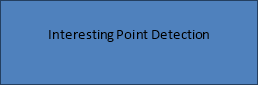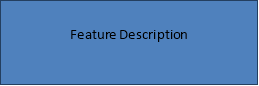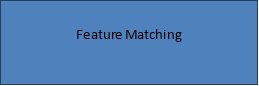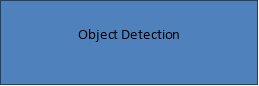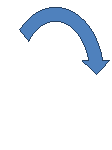Figure3.1: showing the flow chart of the process for object detection

Step 2:

Point Detection:

During the detection step, the local maxima in the box-space of the “determinant of Hessian” operator are used to select interest point candidates. These candidates are then validated if the response is above a given threshold. Both the scale and location of these candidates are then refined using quadratic fitting. Typically, a few hundred interest points are detected in a megapixel image.

input: image u, integral image U, octave o, level i

output: DoHL(u)

function Determinant_of_Hessian (U; o; i)

L 2oi + 1 (Scale variable, Eq. (19))

for x := 0 to M ô€€€ 1, step 2oô€€€1 do (Loop on columns)

for y := 0 to N ô€€€ 1, step 2oô€€€1 do (Loop on rows)

DoHL(u)(x; y) Formula (24) (with (4), (10) and (11))

end for

end for

return DoHL(u)

end function

Algo

input: image u

output: listKeyPoints

(Initialization)

U IntegralImage(u) (Eq. (1))

(Step 1: filtering of features)

for L 2 f3; 5; 7; 9; 13; 17; 25; 33; 49; 65g do (scale sampling)

DoHL(u) Determinant_of_Hessian (U; L)

end for

(Step 2: selection and refinement of keypoints)

for o := 1 to 4 do (octave sampling)

for i := 2 to 3 do (levels sampling for maxima location)

L -> 2o i + 1

listKeyPoints -> listKeyPoints + KeyPoints(o; i;DoHL(u))

end for

end for

return listKeyPoints

So that the scale normalization factor C(L) for second order box filters should be proportional to 1 L2 However, the previous normalization is only true when L1. Indeed, while we have kDxxGσk2 2 kDxyGσk2 2 = 3 at any scale σ, this is not exactly true with box filters, where: kDL xxk2 2 kDL xyk2 2 = 3(2L−1) 2L ≈ 3 when L1. To account for this diï¬€erence in normalization for small scales, while keeping the same (fast) un-normalized box filters, the author of SURF introduced in Formula (24) a weight factor: w(L) = kDL xxk2 kDL xyk2 ·kDxyGσk2 kDxxGσk2 =r2L−1 2L . (26) The numerical values of this parameter are listed in the last column of Table 2. As noticed by the authors of SURF, the variable w(L) does not vary so much across scales. This is the resaon why the weighting parameter w in Eq. (10) is fixed to w(3) = 0.9129.

Feature selection:

In our methodology, interest points are defined as local maxima of the aforementioned DoHL operator applied to the image u. These maxima are detected by considering a 3 × 3 × 3 neighborhood, andperforminganexhaustivecomparisonofeveryvoxelofthediscretebox-spacewith its 26 nearest-neighbors. The corresponding feature selection procedure is described in Algorithm 3.

Algorithm 3

Selection of features

input: o,i,DoHL(u) (Determinant of Hessian response at octave o and level i)

output: listKeyPoints (List of keypoints in box space with sub-pixel coordinates (x,y,L))

function KeyPoints (o,i,DoHL(u)) L ← 2oi + 1 for x := 0 to M −1,

step 2o−1 do (Loop on columns) for y = 0 to N −1, step 2o−1 do (Loop on rows)

if DoHL(u)(x,y) > tH

then (Thresholding)

if isMaximum (DoHL(u),x,y)

then (Non-maximum suppression)

if isRefined (DoHL(u),x,y,L)

end if

end if

end if

end for

end for

return listKeyPoints

end function

Remark A faster method has been proposed in  to find the local maxima without exhaustive search, which has been not implemented for the demo.

Thresholding:

Using four octaves and two levels for analysis, eight diï¬€erent scales are therefore analyzed (see Table 2 in Section 3.2). In order to obtain a compact representation of the image -and also to cope with noise perturbation- the algorithm selects the most salient features from this set of local maxima. This is achieved by using a threshold tH on the response of the DoHL operator DoHL(u)(x,y) > tH . (27) Note that, since the operator is scale-normalized, the threshold is constant. In the demo, this threshold has been set to 10 assuming that the input image u takes values in the intervalJ0,255K. This setting enables us to have a performance similar to the original SURF algorithm [2, 1] (see Section 6 for more details). Figure 13 shows the set of interest points detected as local box-space maxima of the DoHL operator, and selected after thresholding. For visualization purpose, the radii of the circles is set as 2.5 times the box scale L of the corresponding interest points.

View all

## DMCA / Removal Request

If you are the original writer of this essay and no longer wish to have your work published on UKEssays.com then please:

Related Services

Prices from

OMR57

Approximate costs for:

• 1000 words
• 7 day deliveryHumanity University

Dedicated to your worth and value as a human being!

Related Lectures